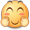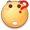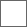• 15回复贴，共1

【教程帖】新课标：口算MD5

Message Digest Algorithm MD5（中文名为消息摘要算法第五版）为计算机安全领域广泛使用的一种散列函数，用以提供消息的完整性保护。该算法的文件号为RFC 1321（R.Rivest,MIT Laboratory for Computer Science and RSA Data Security Inc. April 1992）。

MD5即Message-Digest Algorithm 5（信息-摘要算法5），用于确保信息传输完整一致。是计算机广泛使用的杂凑算法之一（又译摘要算法、哈希算法），主流编程语言普遍已有MD5实现。将数据（如汉字）运算为另一固定长度值，是杂凑算法的基础原理，MD5的前身有MD2、MD3和MD4。

MD5算法具有以下特点：

1、压缩性：任意长度的数据，算出的MD5值长度都是固定的。

2、容易计算：从原数据计算出MD5值很容易。

3、抗修改性：对原数据进行任何改动，哪怕只修改1个字节，所得到的MD5值都有很大区别。

4、强抗碰撞：已知原数据和其MD5值，想找到一个具有相同MD5值的数据（即伪造数据）是非常困难的。

MD5的作用是让大容量信息在用数字签名软件签署私人密钥前被"压缩"成一种保密的格式（就是把一个任意长度的字节串变换成一定长的十六进制数字串）。除了MD5以外，其中比较有名的还有sha-1、RIPEMD以及Haval等。我宋南大哥的帖子没人暖？暖暖暖

_(:зゝ∠)_

md5还能伪造？这是口算吗？

lz就会瞎水贴在MD5算法中，首先需要对信息进行填充，使其字节长度对512求余的结果等于448。因此，信息的字节长度（Bits Length）将被扩展至N*512+448，即N*64+56个字节（Bytes），N为一个正整数。填充的方法如下，在信息的后面填充一个1和无数个0，直到满足上面的条件时才停止用0对信息的填充。然后，在在这个结果后面附加一个以64位二进制表示的填充前信息长度。经过这两步的处理，现在的信息字节长度=N*512+448+64=(N+1)*512，即长度恰好是512的整数倍。这样做的原因是为满足后面处理中对信息长度的要求。
MD5中有四个32位被称作链接变量（Chaining Variable）的整数参数，他们分别为：A=0x01234567，B=0x89abcdef，C=0xfedcba98，D=0x76543210 。
当设置好这四个链接变量后，就开始进入算法的四轮循环运算。循环的次数是信息中512位信息分组的数目。
将上面四个链接变量复制到另外四个变量中：A到a，B到b，C到c，D到d。
主循环有四轮（MD4只有三轮），每轮循环都很相似。第一轮进行16次操作。每次操作对a、b、c和d中的其中三个作一次非线性函数运算，然后将所得结果加上第四个变量，文本的一个子分组和一个常数。再将所得结果向右环移一个不定的数，并加上a、b、c或d中之一。最后用该结果取代a、b、c或d中之一。
以一下是每次操作中用到的四个非线性函数（每轮一个）。
F(X,Y,Z) =(X&Y)|((~X)&Z)
G(X,Y,Z) =(X&Z)|(Y&(~Z))
H(X,Y,Z) =X^Y^Z
I(X,Y,Z)=Y^(X|(~Z))
（&是与，|是或，~是非，^是异或）
这四个函数的说明：如果X、Y和Z的对应位是独立和均匀的，那么结果的每一位也应是独立和均匀的。
F是一个逐位运算的函数。即，如果X，那么Y，否则Z。函数H是逐位奇偶操作符。
假设Mj表示消息的第j个子分组（从0到15），<<
FF(a, b, c, d, Mj, s, ti)表示 a = b + ((a + (F(b, c, d) + Mj + ti) << s
GG(a, b, c, d, Mj, s, ti)表示 a = b + ((a + (G(b, c, d) + Mj + ti) << s
HH(a, b, c, d, Mj, s, ti)表示 a = b + ((a + (H(b, c, d) + Mj + ti) << s
II(a, b, c, d, Mj, s, ti)表示 a = b + ((a + (I(b, c, d) + Mj + ti) << s
这四轮（64步）是：
第一轮
FF(a, b, c, d, M0, 7, 0xd76aa478)
FF(d, a, b, c, M1, 12, 0xe8c7b756)
FF(c, d, a, b, M2, 17, 0x242070db)
FF(b, c, d, a, M3, 22, 0xc1bdceee)
FF(a, b, c, d, M4, 7, 0xf57c0faf)
FF(d, a, b, c, M5, 12, 0x4787c62a)
FF(c, d, a, b, M6, 17, 0xa8304613 )
FF(b, c, d, a, M7, 22, 0xfd469501)
FF(a, b, c, d, M8, 7, 0x698098d8)
FF(d, a, b, c, M9, 12, 0x8b44f7af)
FF(c, d, a, b, M10, 17, 0xffff5bb1)
FF(b, c, d, a, M11, 22, 0x895cd7be)
FF(a, b, c, d, M12, 7, 0x6b901122)
FF(d, a, b, c, M13, 12, 0xfd987193)
FF(c, d, a, b, M14, 17, 0xa679438e)
FF(b, c, d, a, M15, 22, 0x49b40821)

GG(a, b, c, d, M1, 5, 0xf61e2562)
GG(d, a, b, c, M6, 9, 0xc040b340)
GG(c, d, a, b, M11, 14, 0x265e5a51)
GG(b, c, d, a, M0, 20, 0xe9b6c7aa)
GG(a, b, c, d, M5, 5, 0xd62f105d)
GG(d, a, b, c, M10, 9, 0x02441453)
GG(c, d, a, b, M15, 14, 0xd8a1e681)
GG(b, c, d, a, M4, 20, 0xe7d3fbc8)
GG(a, b, c, d, M9, 5, 0x21e1cde6)
GG(d, a, b, c, M14, 9, 0xc33707d6)
GG(c, d, a, b, M3, 14, 0xf4d50d87)
GG(b, c, d, a, M8, 20, 0x455a14ed)
GG(a, b, c, d, M13, 5, 0xa9e3e905)
GG(d, a, b, c, M2, 9, 0xfcefa3f8)
GG(c, d, a, b, M7, 14, 0x676f02d9)
GG(b, c, d, a, M12, 20, 0x8d2a4c8a)
第三轮
HH(a, b, c, d, M5, 4, 0xfffa3942)
HH(d, a, b, c, M8, 11, 0x8771f681)
HH(c, d, a, b, M11, 16, 0x6d9d6122)
HH(b, c, d, a, M14, 23, 0xfde5380c)
HH(a, b, c, d, M1, 4, 0xa4beea44)
HH(d, a, b, c, M4, 11, 0x4bdecfa9)
HH(c, d, a, b, M7, 16, 0xf6bb4b60)
HH(b, c, d, a, M10, 23, 0xbebfbc70)
HH(a, b, c, d, M13, 4, 0x289b7ec6)
HH(d, a, b, c, M0, 11, 0xeaa127fa)
HH(c, d, a, b, M3, 16, 0xd4ef3085)
HH(b, c, d, a, M6, 23, 0x04881d05)
HH(a, b, c, d, M9, 4, 0xd9d4d039)
HH(d, a, b, c, M12, 11, 0xe6db99e5)
HH(c, d, a, b, M15, 16, 0x1fa27cf8)
HH(b, c, d, a, M2, 23, 0xc4ac5665)
第四轮
II(a, b, c, d, M0, 6, 0xf4292244)
II(d, a, b, c, M7, 10, 0x432aff97)
II(c, d, a, b, M14, 15, 0xab9423a7)
II(b, c, d, a, M5, 21, 0xfc93a039)
II(a, b, c, d, M12, 6, 0x655b59c3)
II(d, a, b, c, M3, 10, 0x8f0ccc92)
II(c, d, a, b, M10, 15, 0xffeff47d)
II(b, c, d, a, M1, 21, 0x85845dd1)
II(a, b, c, d, M8, 6, 0x6fa87e4f)
II(d, a, b, c, M15, 10, 0xfe2ce6e0)
II(c, d, a, b, M6, 15, 0xa3014314)
II(b, c, d, a, M13, 21, 0x4e0811a1)
II(a, b, c, d, M4, 6, 0xf7537e82)
II(d, a, b, c, M11, 10, 0xbd3af235)
II(c, d, a, b, M2, 15, 0x2ad7d2bb)
II(b, c, d, a, M9, 21, 0xeb86d391)
常数ti可以如下选择：
在第i步中，ti是4294967296*abs(sin(i))的整数部分，i的单位是弧度。(4294967296等于2的32次方)
所有这些完成之后，将A、B、C、D分别加上a、b、c、d。然后用下一分组数据继续运行算法，最后的输出是A、B、C和D的级联。
当你按照我上面所说的扫二维码下载贴吧客户端